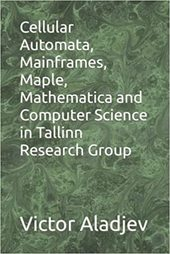# Cellular Automata, Mainframes, Maple, Mathematica and Computer Science in Tallinn Research Group

##### 2022 г.

TRG8090

размещено: 27 Апреля 2022The book presents an expanded excursion into the creative activities of the Tallinn Research Group during 1970–2022 with a focus on researches and development in such main fields as mainframes, personal computers, general statistics theory, issues of parallel information processing and computing, computer mathematics systems (Maple, Mathematica), programming, automated control systems, mathematical developmental biology, some mathematical problems in the cellular automata theory with a focus on our standpoint on the main stages of formation of the cellular automata theory. The book may be of certain interest as a retrospective standpoint on development of the above areas in the USSR (primarily in the ESSR) and the republics of the former USSR. The book is equipped with a fairly extensive bibliography on the issues under consideration.

### Оглавление

INTRODUCTION
CHAPTER 1: Mainframes and Personal Computers
1.1. System and applied programming on mainframes
1.2. System and applied programming on personal computers
1.3. Special courses and books edition on computers
CHAPTER 2: Computer Mathematics Systems
2.1. Background of interest in these issues
2.2. Computer mathematics system Mathematica
2.3. Computer mathematics system Maple
CHAPTER 3: Mathematical Developmental Biology
3.1. General prerequisites
3.2. Discrete modeling in developmental biology
3.3. The French Flag Problem
3.4. The Limited Growth Problem
CHAPTER 4: Certain Mathematical Problems
4.1. The H. Steinhaus combinatorial problem
4.2. The S. Ulam problem from number theory
4.3. An algebraic system for polynomial representation of the a–valued logical functions
CHAPTER 5: Cellular Automata (Homogeneous Structures)
5.1. Basic concepts of classical cellular automata
5.2. The nonсonstructability problem in classical cellular automata
5.3. Extreme design capabilities of classical cellular automata
5.4. The complexity problem of finite configurations in classical cellular automata
5.5. Parallel formal grammars and languages defined by the classical cellular automata (CA–models)
5.6. Modeling problem in classical cellular automata and certain related issues
5.7. The decomposition problem of global transition functions in classical cellular automata (CA–models)
5.8. The main stages of cellular automata theory formation
CONCLUSION
REFERENCES